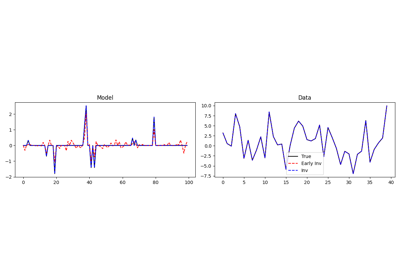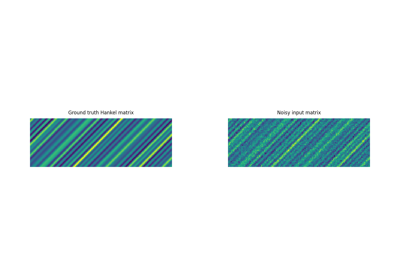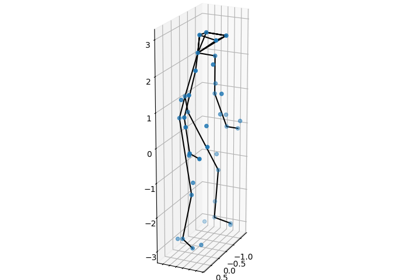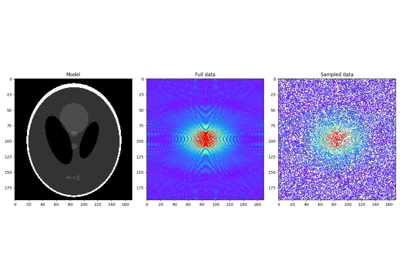pyproximal.optimization.primal.ADMM(proxf, proxg, x0, tau, niter=10, gfirst=False, callback=None, callbackz=False, show=False)[source]#

Alternating Direction Method of Multipliers

Solves the following minimization problem using Alternating Direction Method of Multipliers (also known as Douglas-Rachford splitting):

$\begin{split}\mathbf{x},\mathbf{z} = \argmin_{\mathbf{x},\mathbf{z}} f(\mathbf{x}) + g(\mathbf{z}) \\ s.t. \; \mathbf{x}=\mathbf{z}\end{split}$

where $$f(\mathbf{x})$$ and $$g(\mathbf{z})$$ are any convex function that has a known proximal operator.

ADMM can also solve the problem of the form above with a more general constraint: $$\mathbf{Ax}+\mathbf{Bz}=c$$. This routine implements the special case where $$\mathbf{A}=\mathbf{I}$$, $$\mathbf{B}=-\mathbf{I}$$, and $$c=0$$, as a general algorithm can be obtained for any choice of $$f$$ and $$g$$ provided they have a known proximal operator.

On the other hand, for more general choice of $$\mathbf{A}$$, $$\mathbf{B}$$, and $$c$$, the iterations are not generalizable, i.e. thye depends on the choice of $$f$$ and $$g$$ functions. For this reason, we currently only provide an additional solver for the special case where $$f$$ is a pyproximal.proximal.L2 operator with a linear operator $$\mathbf{G}$$ and data $$\mathbf{y}$$, $$\mathbf{B}=-\mathbf{I}$$ and $$c=0$$, called pyproximal.optimization.primal.ADMML2. Note that for the very same choice of $$\mathbf{B}$$ and $$c$$, the pyproximal.optimization.primal.LinearizedADMM can also be used (and this does not require a specific choice of $$f$$).

Parameters
proxfpyproximal.ProxOperator

Proximal operator of f function

proxgpyproximal.ProxOperator

Proximal operator of g function

x0numpy.ndarray

Initial vector

taufloat, optional

Positive scalar weight, which should satisfy the following condition to guarantees convergence: $$\tau \in (0, 1/L]$$ where L is the Lipschitz constant of $$\nabla f$$.

niterint, optional

Number of iterations of iterative scheme

gfirstbool, optional

Apply Proximal of operator g first (True) or Proximal of operator f first (False)

callbackcallable, optional

Function with signature (callback(x)) to call after each iteration where x is the current model vector

callbackzbool, optional

Modify callback signature to (callback(x, z)) when callbackz=True

showbool, optional

Display iterations log

Returns
xnumpy.ndarray

Inverted model

znumpy.ndarray

Inverted second model

ADMML2

LinearizedADMM

Notes

The ADMM algorithm can be expressed by the following recursion:

$\begin{split}\mathbf{x}^{k+1} = \prox_{\tau f}(\mathbf{z}^{k} - \mathbf{u}^{k})\\ \mathbf{z}^{k+1} = \prox_{\tau g}(\mathbf{x}^{k+1} + \mathbf{u}^{k})\\ \mathbf{u}^{k+1} = \mathbf{u}^{k} + \mathbf{x}^{k+1} - \mathbf{z}^{k+1}\end{split}$

Note that x and z converge to each other, however if iterations are stopped too early x is guaranteed to belong to the domain of f while z is guaranteed to belong to the domain of g. Depending on the problem either of the two may be the best solution.

## Examples using pyproximal.optimization.primal.ADMM#Basis Pursuit

Basis PursuitHankel matrix estimation

Hankel matrix estimationNon-rigid structure-from-motion (NRSfM)

Non-rigid structure-from-motion (NRSfM)Nonlinear inversion with box constraints

Nonlinear inversion with box constraintsPlug and Play Priors

Plug and Play Priors